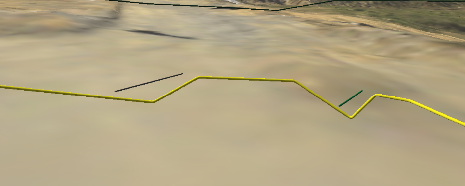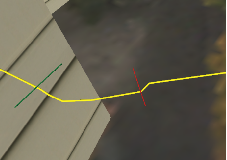# how to create smooth dem and lines from points without changing the vertex number?

1432
8
01-11-2018 04:23 AMNew Contributor

Hi,

I have points and vertices with z values. I want to create smooth lines and smooth dem. However, my each points represent a value which must not be changed.Therefore, I should not create extra point between each point. How can I give this smooth display?

1 Solution

Accepted SolutionsbyEsri Esteemed Contributor

I get your point, the Interpolate Shape—Help | ArcGIS Desktop  tool creates a 3D polyline and inserts vertices using the sample distance which normally corresponds to the cellsize of the DEM. This will create the line that follows the surface and does not respect the rule that only at vertices of the line should be taken into consideration.

The way to resolve this, is using some Python code. The code below will iterate all the vertices of the line and create a 3D polyline featureclass (maintaining all the attributes) and convert the vertices to 3D using the elevation from the DEM/DSM:

``````import arcpy

def main():
import os
arcpy.env.overwriteOutput = True

fc_in = r'C:\GeoNet\Polyline3D\data.gdb\my2Dpipeline'
fc_out = r'C:\GeoNet\Polyline3D\data.gdb\my3Dresult'
dem = r'C:\GeoNet\Polyline3D\data.gdb\DEM'
z_offset = 2

# create output featureclass
sr = arcpy.Describe(fc_in).spatialReference
ws, fc_name = os.path.split(fc_out)
arcpy.CreateFeatureclass_management(ws, fc_name, "POLYLINE", fc_in, "DISABLED", "ENABLED", sr)

# get fields
flds = getFields(fc_in)

# start insert and search cursors
with arcpy.da.InsertCursor(fc_out, flds) as curs_out:
with arcpy.da.SearchCursor(fc_in, flds) as curs:
for row in curs:
pnts_3d = []
polyline_in = row
for part in polyline_in:
for pnt in part:
z = getValueFromDEM(dem, pnt)
pnt_3d = arcpy.Point(pnt.X, pnt.Y, z + z_offset)
pnts_3d.append(pnt_3d)
polyline_out = arcpy.Polyline(arcpy.Array(pnts_3d), sr, True, False)
row_out = list(row)
row_out = polyline_out
row_out = tuple(row_out)
curs_out.insertRow(row_out)

def getValueFromDEM(dem, pnt):
pnt_txt = "{0} {1}".format(pnt.X, pnt.Y)
result = arcpy.GetCellValue_management(dem, pnt_txt)
elevation = float(result.getOutput(0).replace(',', '.'))
return elevation

def getFields(fc):
flds = [fld.name for fld in arcpy.ListFields(fc)]
fld_shp = arcpy.Describe(fc).ShapeFieldname
flds.remove(fld_shp)
flds.insert(0, 'SHAPE@')
return flds

if __name__ == '__main__':
main()
‍‍‍‍‍‍‍‍‍‍‍‍‍‍‍‍‍‍‍‍‍‍‍‍‍‍‍‍‍‍‍‍‍‍‍‍‍‍‍‍‍‍‍‍‍‍‍‍‍‍‍‍``````

Below a 3D view of the result:

• the red line is the result of the interpolate shape tool
• the blue line is the result of the script
• the black points are the vertices of the input line.8 RepliesbyMVP Legendary Contributor

spline interpolation is one option.  interpolation doesn't produce other points, it produces a raster.

Your interpolation's smoothness is only going to be as smooth as the surface you are trying to interpolate and how well you have gathered points so that the interpolation reflects it.

If you sample poorly, then don't expect great results.New Contributor

Hi Dan,

Thank you for your reply. To explain the problem in detailed, I am working with natural gas pipe lines. I take the z values from the surface DEM. I must display my lines smooth and their own vertices. Therefore I need smooth DEM I think. This method that you suggest is suitable for me?byMVP Legendary Contributor

You won't know until you run an interpolation.  Smoothing interpolatants like spline, will not necessarily return the actual value at the observation point (overshoot and undershoot).  You have to decide which is more important and there are dozens of methods of interpolation applicable to their own situations.  There is no one, perfect interpolator.  Experiment once you understand your databyEsri Esteemed Contributor

In case you have a gas line, the vertices should represent the parts of the construction where for instance the line changes in direction (XY) of elevation (Z). When you extract the Z values at the vertices intermediate (virtual) points should not have an elevation to make the line go straight between the defined vertices. It should not be necessary to smooth the DEM in case the DEM represents correctly the surface.

In your resulting line, do you have additional intermediate points with elevation? These may create an incorrect representation of the line. Is it a design of the gas line or the as built?New Contributor

Hi Xander,

I have the datas without their z values and DEM. Firstly, I gave z values by interpolating DEM surface and pull down the gas lines under the surface according to their default or spesific depth. This method gave me lines which are faulted. I illustrated these with images. The yellow lines represent gas pipe lines. The lines must go on smoothly but I should not create extra vertices because of other infrastructure elements.byEsri Esteemed Contributor

I get your point, the Interpolate Shape—Help | ArcGIS Desktop  tool creates a 3D polyline and inserts vertices using the sample distance which normally corresponds to the cellsize of the DEM. This will create the line that follows the surface and does not respect the rule that only at vertices of the line should be taken into consideration.

The way to resolve this, is using some Python code. The code below will iterate all the vertices of the line and create a 3D polyline featureclass (maintaining all the attributes) and convert the vertices to 3D using the elevation from the DEM/DSM:

``````import arcpy

def main():
import os
arcpy.env.overwriteOutput = True

fc_in = r'C:\GeoNet\Polyline3D\data.gdb\my2Dpipeline'
fc_out = r'C:\GeoNet\Polyline3D\data.gdb\my3Dresult'
dem = r'C:\GeoNet\Polyline3D\data.gdb\DEM'
z_offset = 2

# create output featureclass
sr = arcpy.Describe(fc_in).spatialReference
ws, fc_name = os.path.split(fc_out)
arcpy.CreateFeatureclass_management(ws, fc_name, "POLYLINE", fc_in, "DISABLED", "ENABLED", sr)

# get fields
flds = getFields(fc_in)

# start insert and search cursors
with arcpy.da.InsertCursor(fc_out, flds) as curs_out:
with arcpy.da.SearchCursor(fc_in, flds) as curs:
for row in curs:
pnts_3d = []
polyline_in = row
for part in polyline_in:
for pnt in part:
z = getValueFromDEM(dem, pnt)
pnt_3d = arcpy.Point(pnt.X, pnt.Y, z + z_offset)
pnts_3d.append(pnt_3d)
polyline_out = arcpy.Polyline(arcpy.Array(pnts_3d), sr, True, False)
row_out = list(row)
row_out = polyline_out
row_out = tuple(row_out)
curs_out.insertRow(row_out)

def getValueFromDEM(dem, pnt):
pnt_txt = "{0} {1}".format(pnt.X, pnt.Y)
result = arcpy.GetCellValue_management(dem, pnt_txt)
elevation = float(result.getOutput(0).replace(',', '.'))
return elevation

def getFields(fc):
flds = [fld.name for fld in arcpy.ListFields(fc)]
fld_shp = arcpy.Describe(fc).ShapeFieldname
flds.remove(fld_shp)
flds.insert(0, 'SHAPE@')
return flds

if __name__ == '__main__':
main()
‍‍‍‍‍‍‍‍‍‍‍‍‍‍‍‍‍‍‍‍‍‍‍‍‍‍‍‍‍‍‍‍‍‍‍‍‍‍‍‍‍‍‍‍‍‍‍‍‍‍‍‍``````

Below a 3D view of the result:

• the red line is the result of the interpolate shape tool
• the blue line is the result of the script
• the black points are the vertices of the input line.New Contributor

Hi Xander,

Thank you for your help. It is the result that I want about smoothing the line in their depth (z value) perspective. Thanks a lot again.byEsri Esteemed Contributor

You're welcome. Please test the procedure and if you have any problems, just post them back here. If you encounter no problems, please mark the post as  correct answer.